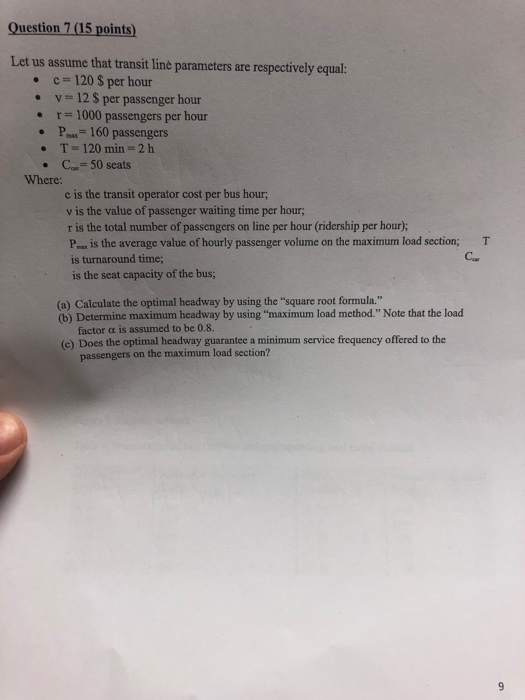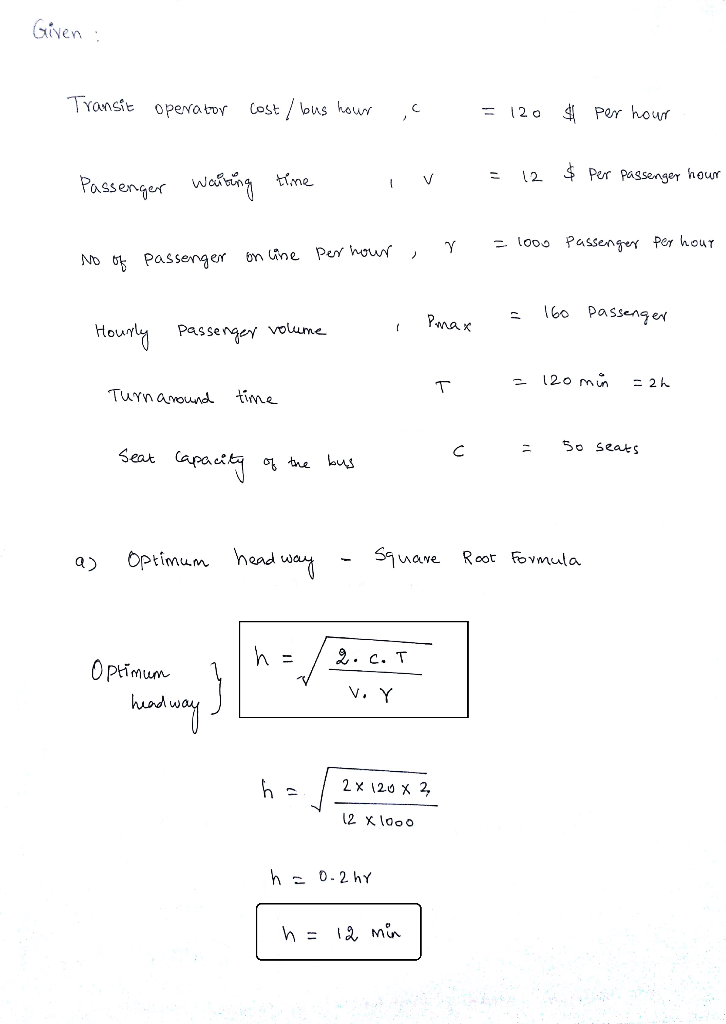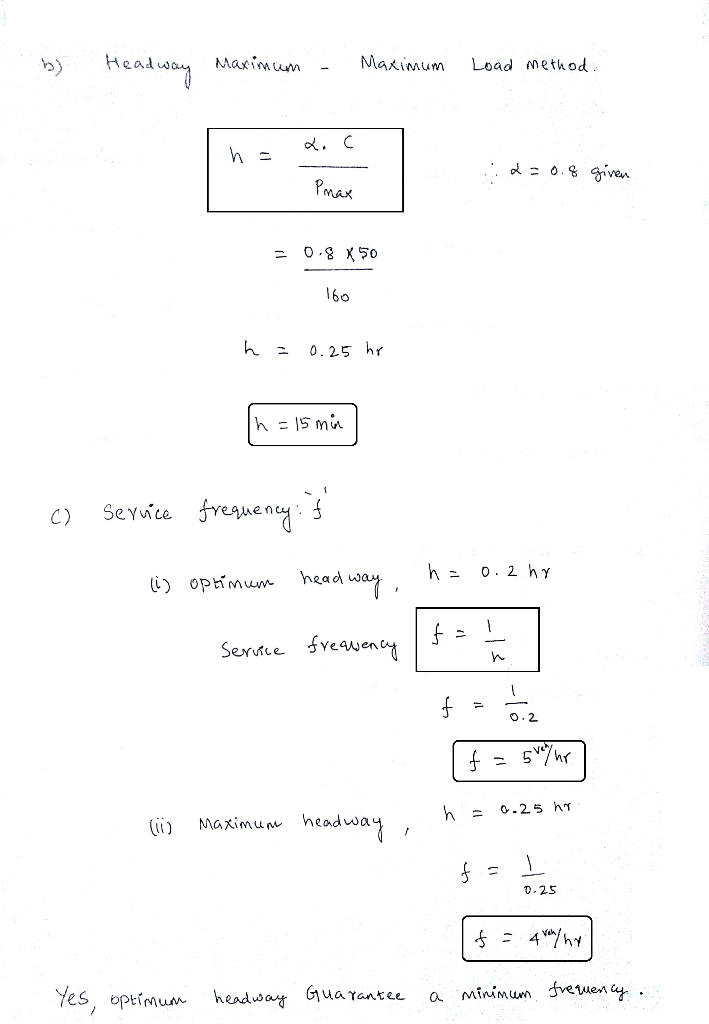# Transportation Engineering: Question 7 (15 points) Let us assume that transit line parameters are respectively equal: c 120 S per hour v-12 S per passenger hour . r-1000 passengers per hour P-...

Transportation Engineering:Question 7 (15 points) Let us assume that transit line parameters are respectively equal: c 120 S per hour v-12 S per passenger hour . r-1000 passengers per hour P- 160 passengers T- 120 min- 2 h C-50 seats Where: c is the transit operator cost per bus hour; v is the value of passenger waiting time per hour r is the total number of passengers on line per hour (ridership per hour); P is the average value of hourly passenger volume on the maximum load section; T is turnaround time; is the seat capacity of the bus; (a) Calculate the optimal headway by using the "square root formula." (b) Determine maximum headway by using "maximum load method." Note that the load factor a is assumed to be 0.8. (c) Does the optimal headway guarantee a minimum service frequency offered to the passengers on the maximum load section?##### Add Answer of: Transportation Engineering: Question 7 (15 points) Let us assume that transit line parameters are respectively equal: c 120 S per hour v-12 S per passenger hour . r-1000 passengers per hour P-...
Similar Homework Help Questions
Free Homework App